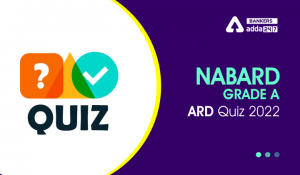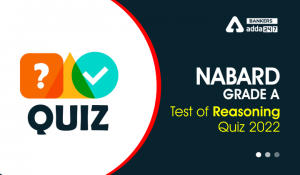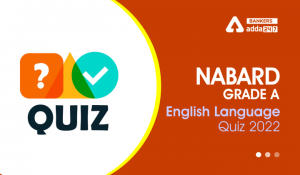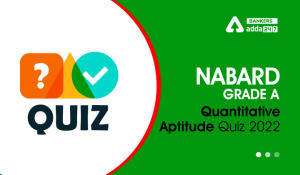Latest Banking jobs   »

# Reasoning Ability Quiz For NABARD Grade A 2022- 26th July

Directions (1-3): Study the numbers and letters sequence carefully and answer the questions given below.

X 7 A 0 8 S 9 T 1 4 U 5 0 Q 6 Z 3 2 C 1 8 V 5 B 2 E N 9 6 M 0 3 O 7 F 4 1 I

Q1. Which amongst the following element is 16th to the left of 28th element from the left end?
(a) V
(b) 5
(c) B
(d) 2
(e) None of these

Q2. How many elements are there between ‘Q’ and the one which is second to the right of ‘N’?
(a) Fourteen
(b) Fifteen
(c) Thirteen
(d) Sixteen
(e) None of these

Q3. What is the product of the number which is 4th number from the left end and 7th number from the right end (exclude all the alphabets while counting numbers)?
(a) 48
(b) 18
(c) 81
(d) 54
(e) None of these

Directions (4-7): These questions are based on the following arrangement.

W R – 8 & M P 9 W C N 2 + = X * A 0 % Z Y \$ 1 # 5 K I 4

Q4. How many such symbols are there in the above arrangement which are immediately followed by a letter as well as immediately preceded by a number?
(a) Two
(b) None
(c) Four
(d) Three
(e) None of these

Q5. Which element is exactly between 6th element from right and 11th element from left end?
(a) A
(b) X
(c) %
(d) Z
(e) None of these

Q6. What is the sum of 3rd number from right end and 2nd number from left end?
(a) 12
(b) 8
(c) 15
(d) 10
(e) None of these

Q7. If W R – 8 are written in the reverse order, & M P 9 are written in the reverse order and so on, then in the new arrangement which of following will be exactly between 2 and \$?
(a) X
(b) +
(c) =
(d) *
(e) None of these

Directions (8-12): Study the following data carefully and answer the questions accordingly.

7 8 5 3 8 8 9 2 4 5 2 1 4 3 7 6 6 8 9 5 7 2 5 4 7 8 6 2 5

Q8. How many even numbers that are immediately preceded by an even number and immediately followed by an odd number?
(a) Two
(b) More than three
(c) Three
(d) One
(e) None

Q9. What is the difference between the sum of the digits that are 4th, 6th and 11th from the left end and 4th, 6th and 11th from the right end?
(a) 10
(b) 7
(c) 12
(d) 8
(e) None of these

Q10. How many odd numbers that are immediately preceded by an even number?
(a) Eighteen
(b) Twelve
(c) Nine
(d) Seventeen
(e) None of these

Q11. Which of the following digit is exactly between the one which is 20th from right end and which is 8th from left end?
(a) 8
(b) 5
(c) 2
(d) 4
(e) None of these

Q12. Which of the following digit is 13th to the right of the one which is 15th from the left end?
(a) 7
(b) 2
(c) 6
(d) 5
(e) None of these

Directions (13-15): Read the following alphanumeric series carefully and answer the questions given below.

4 P J 3 % T @ U K 5 V 1 W # Y 2 B 6 & 9 D 8 G © Z

Q13. Which of the following is the sixth to the left of the fifteenth from the left end of the given arrangement?
(a) 2
(b) &
(c) #
(d) K

Q14. How many such numbers are there in the given arrangement, each of which is immediately preceded by a consonant and immediately followed by a symbol?
(a) None
(b) One
(c) Two
(d) Three
(e) More than three

Q15. If the first five elements and the last ten elements are written in the reverse order, which of the following will be the seventh element to the right of the eleventh element from your left?
(a) %
(b) G
(c) Y
(d) 2
(e) B

Solutions

S1. Ans.(b)
Sol. Clearly, 5 is 16th to the left of 28th element from the left end.

S2. Ans.(a)
Sol. Clearly, fourteen elements are there between ‘Q’ and the one which is second to the right of ‘N’

S3. Ans.(c)
Sol. 4th number from the left end = 9
6th number from the right end = 9
So, product of these numbers = 9×9=81.

S4. Ans.(a)
Sol. Clearly, two symbols (8&M, 0%Z) are there in the above arrangement.

S5. Ans.(a)
Sol. Clearly, A is exactly between 6th element from right and 11th element from left end.

S6. Ans.(d)
Sol. 3rd number from right end = 1
2nd number from left end = 9
So, sum of these number = 1+9 = 10.

S7 Ans.(b):
Sol. Clearly, + will be exactly between 2 and \$.

S8. Ans.(b)
Sol. Clearly, there are four numbers (889, 245,689, 625).

S9. Ans.(d)
Sol. Clearly, 8 is the difference between the sum of the digits.

S10. Ans.(c)

S11. Ans.(d)
Sol. Clearly, digit 4 is exactly between the one which is 20th from right end and which is 8th from left end.

S12. Ans.(b)
Sol. Clearly, digit 2 is 13th to the right of the one which is 15th from the left end.

S13. Ans.(d)
Sol. Clearly, K is the sixth to the left of the fifteenth from the left end.

S14. Ans.(c)
Sol. Clearly, there are two numbers which is immediately preceded by a consonant and immediately followed by a symbol.

S15. Ans.(b)
Sol. Clearly, G will be the seventh element to the right of the eleventh element from your left.#### Congratulations!Incorrect details? Fill the form again here

•Agriculture and Rural Development Quizze...
•Reasoning Ability Quiz For NABARD Grade ...
•English Quizzes For NABARD Grade A 2022-...
•Quantitative Aptitude Quiz For NABARD Gr...
•Reasoning Ability Quiz For NABARD Grade ...
•English Quizzes For NABARD Grade A 2022-...